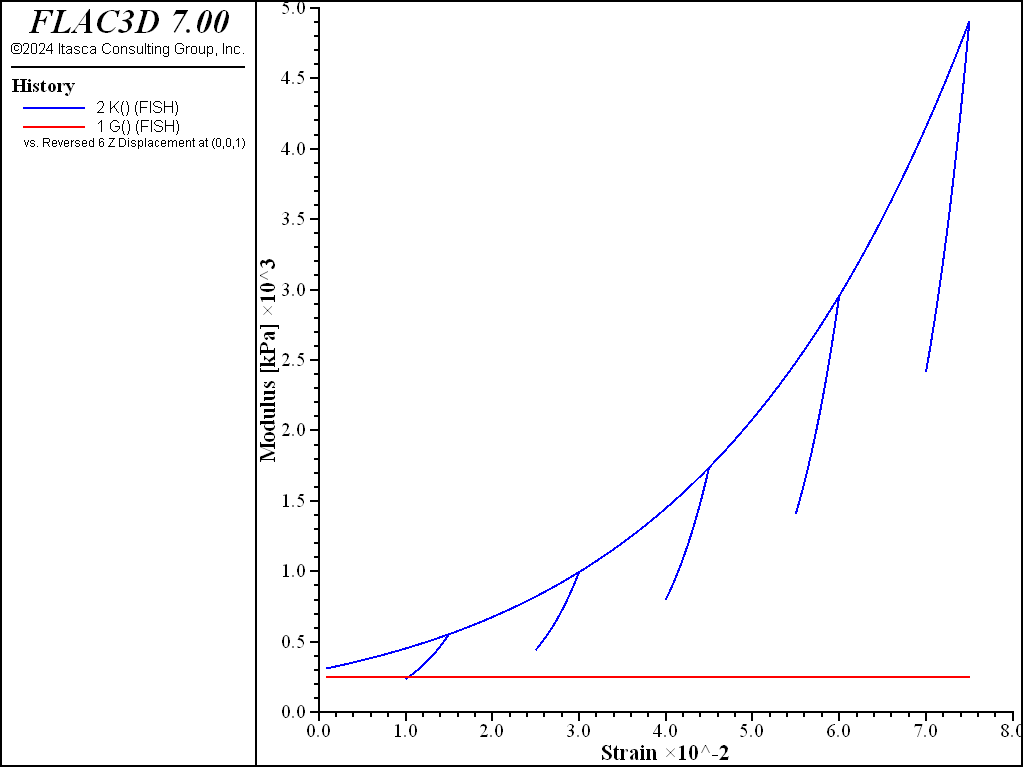FLAC3D Theory and Background • Constitutive Models

# Isotropic Consolidation Test with Modified Cam-Clay Model

Note

The project file for this example is available to be viewed/run in FLAC3D. The project’s main data file is shown at the end of this example.

This simple one-zone example exercises the modified Cam-Clay model for a normally consolidated material subjected to several load-unload excursions in an isotropic compression test. Following sentence doesn’t make sense. The initial stresses is at isotropic stress so that $$\sigma_{xx}$$ = $$\sigma_{yy}$$ = $$\sigma_{zz}$$ = -5 kPa. The material properties include the following:

 maximum shear modulus ($$G_{max}$$) 10 MPa shear modulus ($$G$$) 250 kPa ratio-critical state ($$M$$) 1.02 slope of normal consolidation line ($$\lambda$$) 0.2 slope of elastic swelling line ($$\kappa$$) 0.05 pressure reference ($$p_1$$) 1 kPa specific volume reference ($$v_{\lambda}$$) 3.32 pressure consolidation ($$p_{c0}$$) -5 kPa

The sample is assumed normally isotropic consolidation with $$OCR$$ = 1, so the initial mean effective pressure and the preconsolidation pressure are the same. Their values are specified using a simple FISH function:

fish define camclay_ini_p
loop foreach local p_z zone.list
local mean_p = -(zone.stress.xx(p_z) + zone.stress.yy(p_z) ...
+ zone.stress.zz(p_z))/3.0 - z.pp(p_z)
zone.prop(p_z,'pressure-effective') = mean_p
zone.prop(p_z,'pressure-preconsolidation') = mean_p
endloop
end


The results are shown in Figure 1, 2, and 3. Figure 1 plots the mean effective pressure history. Figure 2 plots the relation $$v$$ vs. $$\ln p$$, which shows the normal consolidation line and elastic swelling lines. In this example, the shear modulus is explicitly specified as an input parameter; it is thus assumed constant, while the bulk modulus is changing with the mean effective pressure. The bulk and shear modulus histories are plotted in Figure 3.Figure 3: Bulk and shear moduli versus displacement.

Data File

IsotropicConsolidationModifiedCamClay.dat

;------------------------------------------------------------
; Isotropic compression test on Modified-Cam-clay sample (drained)
;------------------------------------------------------------
model new
model large-strain off
fish automatic-create off
model title "Isotropic compression test for normally consolidated soil"

zone create brick size 1 1 1
zone cmodel assign modified-cam-clay
; --- boundary and initial conditions ---
zone gridpoint fix velocity
zone initialize stress xx -5. yy -5. zz -5.
; --- model properties ---
zone property shear 250. bulk-maximum 10000.
zone property ratio-critical-state 1.02 lambda 0.2 kappa 0.05
zone property pressure-reference 1. specific-volume-reference 3.32
;
program call 'fishfunctions'
[camclay_ini_p]
; --- histories ---
history interval 20
fish history G
fish history K
fish history p
fish history lnp
fish history v
zone history displacement-z position 0 0 1
; --- test ---